# Class 6 Maths Chapter 6:Fractions Exercise 7.1 and 7.2

In this page we have NCERT Solutions for Class 6 Maths Chapter 6:Fractions Exercise 7.1 and 7.2. Hope you like them and do not forget to like , social share and comment at the end of the page.

## Exercise 7.1

Question 1
Write the fraction representing the shaded portion.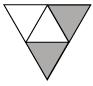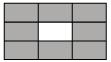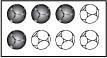(i) (ii) (iii)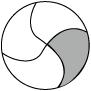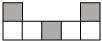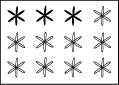(iv) (v) (vi)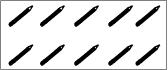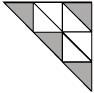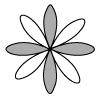(vii) (viii) (ix)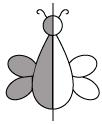(x)
 S.no Answer Reason a) 2/4 There are four equal parts out of which 2 parts are shaded b) 8/9 There are 9 equal parts out of which 8 parts are shaded c) 4/8 There are 8 equal parts out of which 4 parts are shaded d) 1/4 There are 4 equal parts out of which 1 part is shaded e) 3/7 There are 7 equal parts out of which 3 parts are shaded f) 3/12 There are 12 flowers of the same kind out of which 3 are shaded g) 10/10 There are 10 pencils out of which 10 are shaded h) 4/9 There are 9 equal parts out of which 4 parts are shaded i) 4/8 There are 8 equal parts out of which 4 parts are shaded j) 1/2 There is a shape divided into 2 equal parts, out of which one part is shaded
Question 2
Colour the part according to the given fraction.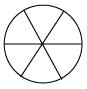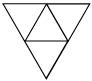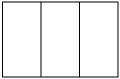(i) 1/6 (ii)1/4 (iii)1/3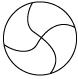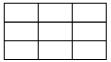(iv) 3/4 (v) 4/9
 i)1/6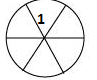ii) 1/4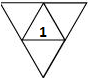iii)1/3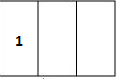iv)3/4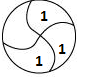v)4/9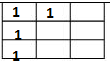Question 3
Identify the error if any.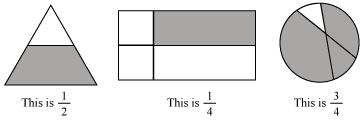The given figures do not represent the correct fractions as the part are unequal
Question 4
What fraction of a day is 8 hours?
We know that in a day there are 24 hours. So, 8 hours in a 24-hour day is represented as 8/24
Question 5
What fraction of an hour is 40 minutes?
We know that in an hour there are 60 minutes. So, 40 minutes in a 60 min is represented as 40/60
Question 6
Arya, Abhimanyu, and Vivek shared lunch. Arya has brought two sandwiches, one made of vegetable and one of jam. The other two boys forgot to bring their lunch. Arya agreed to share his sandwiches so that each person will have an equal share of each sandwich.
(a) How can Arya divide his sandwiches so that each person has an equal share?
(b) What part of a sandwich will each boy receive?
a) Since there are three people to eat, Arya should divide his sandwiches into 3 equal parts and give 1 part of each sandwich so that each person has an equal share.
b)      Each boy will receive 1/3 of a sandwich.
Question 7
Kanchan dyes dresses. She had to dye 30 dresses. She has so far finished 20 dresses. What fraction of dresses has she finished?
Kanchan has finished 20 dresses out of 30. So Fraction would be 20/30
Question 8
Write the natural numbers from 2 to 12. What fraction of them are prime numbers?
Natural numbers from 2 to 12 are 2, 3, 4, 5, 6, 7, 8, 9, 10, 11 and 12
Prime numbers – 2, 3, 5, 7, 11
There are 11 natural numbers from 2 to 12 out of which 5 are prime numbers.
Fraction of numbers that are prime is 5/11
Question 9
Write the natural numbers from 102 to 113. What fraction of them are prime numbers?
Natural numbers from 102 to 113 are 102, 103, 104, 105, 106, 107, 108, 109, 110, 111, 112 and 113
Prime numbers – 103, 107, 109, 113
There are 12 natural numbers from 102 to 113 out of which 4 are prime numbers.
So, fraction of numbers that are prime is 4/12
Question 10
What fractions of these circles have X’s in them?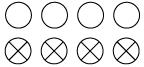There are 8 circles out of which 4 have X’s in them.
So, fraction of circles having X’s is 4/8
Question 11
Kristin received a CD player for her birthday. She bought 3 CDs and received 5 others as gifts. What fraction of her total CDs did she buy and what fraction did she receive as gifts?
Number of CDs Kristin purchased = 3
Number of CDs she received as gifts = 5
So, total number of CDs = 8
Kristin purchased 3 CDs out of a total number of 8 CDs.
Fraction of CDs she purchased = 3/8
Kristin received 5 CDs out of a total number of 8 CDs.
Fraction of CDs she received as gifts =5/8

## Exercise 7.2

Question 1
Draw number lines and locate the points on them:
(a) $\frac {1}{2},\frac {1}{4},\frac {3}{4},\frac {4}{4}$
(b)$\frac {1}{8},\frac {2}{8},\frac {3}{8},\frac {7}{8}$
(c)$\frac {2}{5},\frac {3}{5},\frac {8}{5},\frac {4}{5}$
a)  These fraction lies between 0 and 1 in the number line.  We can divide the distance between 0 and 1 on the number into four equal part, then each point will represent these fractions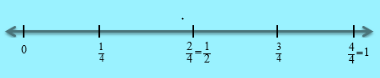b) These fraction lies between 0 and 1 in the number line.  We can divide the distance between 0 and 1 on the number into 8 equal part, then each point will represent these fractions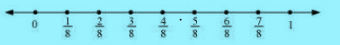c) These fraction lies between 0 and 1 in the number line.  We can divide the distance between 0 and 1 on the number into 5 equal part, then each point will represent these fractions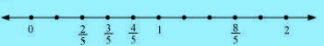Question 2
Express the following as mixed fractions:
(a) 20/3
(b)11/5
(c)17/7
(d)28/5
(e)19/6
(f)35/9
A mixed fraction is a combination of whole and part
To convert an improper fraction to a mixed fraction, follow these steps:
1.Divide the numerator by the denominator.
2.Write down the whole number answer.
3.Then write down any remainder above the denominator.
$6 \frac {2}{3}$
 S.no Question Calculation Answer a) 20/3 (18+2)/3= 6+ 2/3 b) 11/5 (10+1)/5= 2+1/5 $2 \frac {1}{5}$ c) 17/7 (14+3)/7=2 +3/7 $2 \frac {3}{7}$ d) 28/5 (25+3)/5= 5+3/5 $5 \frac {3}{5}$ e) 19/6 (18+1)/6= 3+ 1/6 $3 \frac {1}{6}$ f) 35/9 (27+8)/9= 3+ 8/9 $3 \frac {8}{9}$
Question 3
Express the following as improper fractions:
(a)$7 \frac {3}{4}$
(b)$5 \frac {6}{7}$
(c)$2 \frac {5}{6}$ (d)$10 \frac {3}{5}$
(e)$9 \frac {3}{7}$
(f)$8 \frac {4}{9}$
We can express a mixed fraction as improper fraction in this way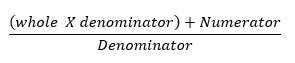S.no Question Calculation Answer a) $7 \frac {3}{4}$ (28+3)/4 31/4 b) $5 \frac {6}{7}$ (35+6)/7 41/7 c) $2 \frac {5}{6}$ (12+5)/6 17/6 d) $10 \frac {3}{5}$ (50+3)/5 53/5 e) $9 \frac {3}{7}$ (63+3)/7 66/7 f) $8 \frac {4}{9}$ (72+4)/9 76/9

### Practice Question

Question 1 What is $\frac {1}{2} + \frac {3}{4}$ ?
A)$\frac {5}{4}$
B)$\frac {1}{4}$
C)$1$
D)$\frac {4}{5}$
Question 2 Pinhole camera produces an ?
A)An erect and small image
B)an Inverted and small image
C)An inverted and enlarged image
D)None of the above